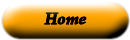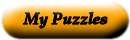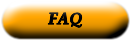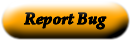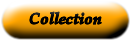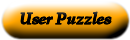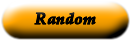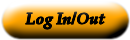### Mathmetic Word Match Puzzle

Jason Morlock

Match each math vocabulary word to its definition on the opposite side.

 Hexagon The value of a variable that makes an equation true. Heptagon A polygon with 8 sides. Horizontal The set of numbers containing zero, the natural numbers, and all the negatives of the natural numbers. Hypotenuse A polygon with 7 sides. Inequality A three-dimensional figure with all points in space a fixed distance from a given point, called the center. Infinity A whole number that is not divisible by 2. Integer The point (0, 0) on a coordinate plane, where the x-axis and the y-axis intersect. Intercept The root of an equation is the same as the solution to the equation. Natural Number A mathematical expression which shows that two quantities are not equal. Negative Number An angle measuring more than 90 degrees. Number Line A real number that is less than zero. Numerator A limitless quantity. Obtuse Angle The x-intercept of a line or curve is the point where it crosses the x-axis, and the y- intercept of a line or curve is the point where it crosses the y-axis. Octagon In statistics, the difference between the largest and the smallest numbers in a data set. Origin A quadrilateral with four equal sides and four 90 degree angles. Odd Number The line opposite the right angle in a ight triangle. Radius The distance from the center to a point on a circle; the line segment from the center to a point on a circle. Range The top part of a fraction. Ratio A pair of numbers that compares different types of units. Root A line with zero slope. Slope A polygon with 6 sides. Solution A line on which every point represents a real number. Sphere The steepness of a line expressed as a ratio, using any two points on the line. Square The counting numbers.

Use the "Printable HTML" button to get a clean page, in either HTML or PDF, that you can use your browser's print button to print. This page won't have buttons or ads, just your puzzle. The PDF format allows the web site to know how large a printer page is, and the fonts are scaled to fill the page. The PDF takes awhile to generate. Don't panic!Web armoredpenguin.com